### Specifying the Number of Knots

Keep the number of knots small (usually less than 10, although you can specify more). A degree-three spline with nine knots, one at each decile, can closely follow a large variety of curves. Each spline transformation of degree p with q knots fits a model with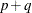parameters. The total number of parameters should be much less than the number of observations. Usually in regression analyses, it is recommended that there be at least five or ten observations for each parameter in order to get stable results. For example, when spline transformations of degree three with nine knots are requested for six variables, the number of observations in the data set should be at least 5 or 10 times 72 (since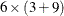is the total number of parameters). The overall model can also have a parameter for the intercept and one or more parameters for each nonspline variable in the model.

Increasing the number of knots gives the spline more freedom to bend and follow the data. Increasing the degree also gives the spline more freedom, but to a lesser extent. Specifying a large number of knots is much better than increasing the degree beyond three.

When you specify NKNOTS=q for a variable with n observations, then each of the q + 1 segments of the spline contains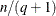observations on the average. When you specify KNOTS=number-list, make sure that there is a reasonable number of observations in each interval.

The following statements find a cubic polynomial transformation of x and no transformation of y:

proc transreg;
model identity(y)=spline(x);
output;
run;


The following statements find a cubic-spline transformation for x that consists of the weighted sum of a single constant, a single straight line, a quadratic curve for the portion of the variable less than 3.0, a different quadratic curve for the portion greater than 3.0 (since the 3.0 knot is repeated), and a different cubic curve for each of the intervals: (minimum to 1.5), (1.5 to 2.4), (2.4 to 3.0), (3.0 to 4.0), and (4.0 to maximum):

proc transreg;
model identity(y)=spline(x / knots=1.5 2.4 3.0 3.0 4.0);
output;
run;


The transformation is continuous everywhere, its first derivative is continuous everywhere, its second derivative is continuous everywhere except at 3.0, and its third derivative is continuous everywhere except at 1.5, 2.4, 3.0, and 4.0.

The following statements find a quadratic spline transformation that consists of a polynomial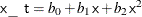for the range (x < 3.0) and a completely different polynomial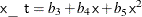for the range (x > 3.0):

proc transreg;
model identity(y)=spline(x / knots=3 3 3 degree=2);
output;
run;


The two curves are not required to be continuous at 3.0.

The following statements categorize y into 10 intervals and find a step-function transformation:

proc transreg;
model identity(y)=spline(x / degree=0 nknots=9);
output;
run;


One aspect of this transformation family is unlike all other optimal transformation families. The initial scaling of the data does not fit the restrictions imposed by the transformation family. This is because the initial variable can be continuous, but a discrete step-function transformation is sought. Zero-degree spline variables are categorized before the first iteration.

The following statements find a continuous, piecewise linear transformation of x:

proc transreg;
model identity(y)=spline(x / degree=1 nknots=8);
output;
run;